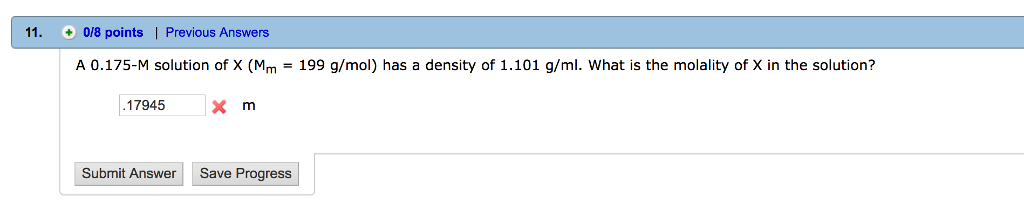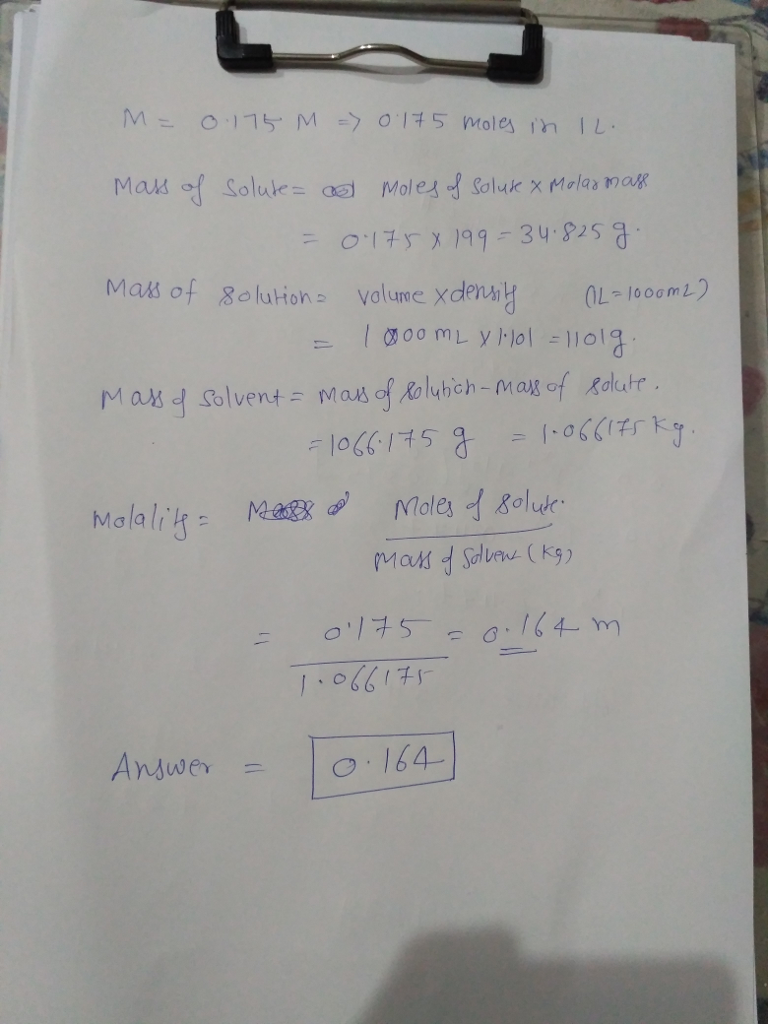# Question & Answer: A 0.175-M solution of X (M_m = 199 g/mol) has a density of 1.101 g/ml. What is the molality of…..A 0.175-M solution of X (M_m = 199 g/mol) has a density of 1.101 g/ml. What is the molality of X in the solution?